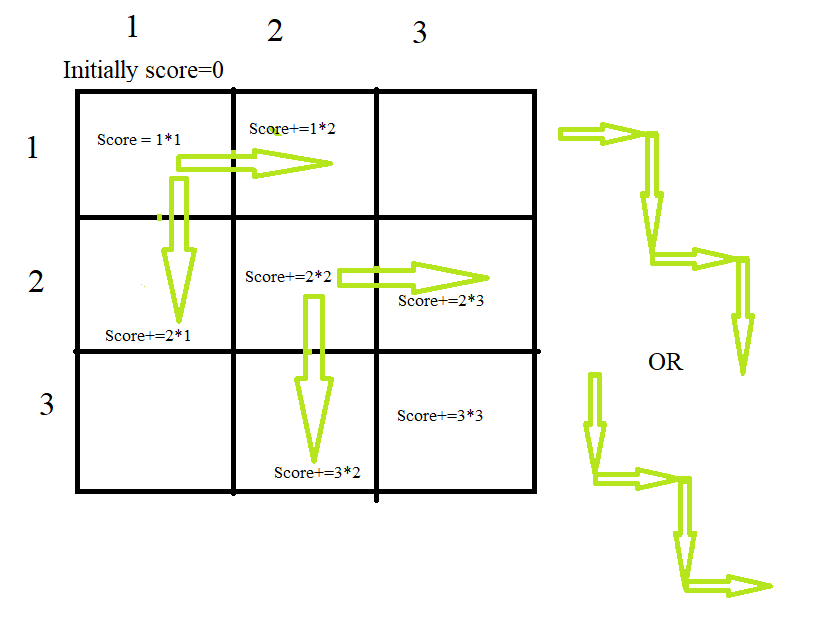GFG App
Open AppBrowser
Continue

# Maximum Score by travelling from (1, 1) to (N, N) on grid

Given an integer N which corresponds to a N x N grid. The score you can get from a cell at the ith row and the jth column is i*j. You are initially at position (1, 1) of the grid and you have to reach (N, N) by making the score as max as possible. The only direction you can move is right (from (i, j) to (i, j + 1)) and down (from (i, j) to (i+1, j)). You are not allowed to go out of the grid. The answer might be a large number so return ans mod 10^9+7.

Examples:

Input: 3
Output: 22
Explanation: 1*1 + 1*2 + 2*2 + 2*3 + 3*3 = 22

Input: 10
Output: 715

Approach: To solve the problem follow the below idea:

You can visualize that the best path is in a zigzag fashion, i.e. from (1, 1) to (1, 2) to (2, 2) and so on. Thus, the answer would be the sum of the product of coordinates from
(1, 1) to (N, N)

(1*1)+(1*2)+(2*2)+…+(N*N)Illustration:Best path for 1*1 to n*n is zigzag traversal

In the above illustration, we are given a 3*3 grid we need to find the best path which is shown in the figure. We have two paths which are best so we can choose any of them.

Below are the steps for the above approach:

• Initialize a variable ans that will store the final answer.
• Use the derived formula to find the answer.
• ans = (((((n * (n + 1)) % mod) * (4 * n – 1)) % mod) / 6) % mod.
• As the answer might be a large number so print ans as (ans % 10^9 + 7).

Below is the implementation for the above approach:

## C++

 // C++ code for the above approach: #include  const int mod = 1e9 + 7; using namespace std; void getScore(long long n) {     long long ans         = (((((n * (n + 1)) % mod) * (4 * n - 1)) % mod)            / 6)           % mod;     cout << ans << endl; }   // Drivers code int main() {     getScore(2);     getScore(4);     getScore(10);     getScore(100); }

## Java

 //Java code for the above approach: import java.util.*;   public class Main {   final static int MOD = (int)1e9 + 7;     public static void getScore(long n) {     long ans = (((((n * (n + 1)) % MOD) * (4 * n - 1)) % MOD) / 6) % MOD;     System.out.println(ans);   }     // Drivers code   public static void main(String[] args) {     getScore(2);     getScore(4);     getScore(10);     getScore(100);   } }

## Python3

 MOD = 10**9 + 7   def getScore(n):     ans = (((((n * (n + 1)) % MOD) * (4 * n - 1)) % MOD) // 6) % MOD     print(ans)   # Drivers code getScore(2) getScore(4) getScore(10) getScore(100)

## C#

 // C# code for the above approach:   using System;   public class GFG {       const int MOD = (int)1e9 + 7;       static void getScore(long n)     {         long ans             = (((((n * (n + 1)) % MOD) * (4 * n - 1)) % MOD)                / 6)               % MOD;         Console.WriteLine(ans);     }       static public void Main()     {           // Code         getScore(2);         getScore(4);         getScore(10);         getScore(100);     } }   // This code is contributed by lokesh.

## Javascript

 const MOD = 1e9 + 7;   function getScore(n) {   const ans = (((n * (n + 1)) % MOD) * ((4 * n) - 1) % MOD) / 6 % MOD;   console.log(ans); }   // Driver code getScore(2); getScore(4); getScore(10); getScore(100);

Output

7
50
715
671650

Time Complexity: O(1)
Auxiliary Space: O(1)

My Personal Notes arrow_drop_up